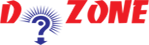﻿ Python Variables & Assignments: Description in Brief## Variables and Assignments - Introduction

### Assignments

An assignment in Python has the form variable = expression. This has the following effect. Firstly the expression on the right side is evaluated, then the result is assigned to the variable. After the assignment is done, the variable becomes a name for the result. The variable remains the same until the another value is assigned, in this case the previous value is lost.

Executing the assignment produces no output; its purpose is to make the association between the variable and its value.

``````
>>> x = 3+3
>>> print x
6
```
```

From the above example, the assignment statement stated that x is equal to 4, producing no output. If you want to see the value of x, we print it. If we execute another assignment to x, then the previous value is lost.

``````
>>> x = 280.5
>>> print x
280.5
>>> y = 2* x
>>> print y
561.0
```
```

Remember: A single "=" equal to is used for assignment, the double "==" equal sign is used to test for equality.

In Maths the equation x = x + 1 is nothing; as it has no solution. Whereas, nn Computer Science, the statement x = x + 1 is very useful. Its purpose is to add 1 to x, and reassign the result to x. In short, x is incremented by 1.

``````
>>> x = 10
>>> x = x + 1
>>> print x
11

>>> x = x + 1
>>> print x
12
```
```

### Variables

Variable are the contiguous sequence of letters, numbers, and the underscore (_) character. The first character should not be a number, and you may not use a reserved word as a variable name. Examples of legal variable names are: a, v1, v_1, abc, Bucket, monthly_total, __pi__, TotalAssets.

## Other Python Programs

PythonTraining, 258-FF , Katewa Nagar, New Sanganer Rd, Jaipur, 302019